Courses

# Test: Enthalpy Of Reaction And Formation

## 18 Questions MCQ Test Chemistry Class 11 | Test: Enthalpy Of Reaction And Formation

Description
This mock test of Test: Enthalpy Of Reaction And Formation for JEE helps you for every JEE entrance exam. This contains 18 Multiple Choice Questions for JEE Test: Enthalpy Of Reaction And Formation (mcq) to study with solutions a complete question bank. The solved questions answers in this Test: Enthalpy Of Reaction And Formation quiz give you a good mix of easy questions and tough questions. JEE students definitely take this Test: Enthalpy Of Reaction And Formation exercise for a better result in the exam. You can find other Test: Enthalpy Of Reaction And Formation extra questions, long questions & short questions for JEE on EduRev as well by searching above.
QUESTION: 1

### Direction (Q. Nos. 1-9) This section contains 9 multiple choice questions. Each question has four choices (a), (b), (c) and (d), out of which ONLY ONE option is correct. Q. The difference between ΔrH° and ΔrE° (in kcal) for the reaction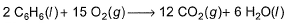at 298 K in kcal is

Solution:

∆rH° =  ∆rE° + ∆ngRT
∆rH° - ∆rE° = ∆ngRT
= (12-15)× 2× 298
= -1.788 kcal

QUESTION: 2

### The ΔrH° for CO2(g), CO(g) , and H2O(g) are - 393.5°, - 110.5 and - 241.8 kJ mol-1, respectively. Thus, ΔrH° for the reaction (in kJ)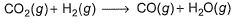[IITJEE 2000]

Solution:

∆H= ∆HCO+∆HH2O-∆HCO2 (as ΔH for H2 is 0)
= -110.5-241.8+393.5 = +41.2 kJ mol-1

QUESTION: 3

### Enthalpies of formation of CO(g) , CO2 (g) , N2O (g) and N2O4 (g) are -110, - 393, 81 and 9.7 kJ mol-1. Thus, ΔrU for the reaction at 298 K is,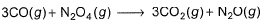Solution:

ΔrH=ΔrH(product)-ΔrH(reactant)
=3×-393+81+3×110-9.7
=-777.7kJ
ΔH = ΔU+ΔngRT
AS Δng = 0, ΔH = ΔU
So, ΔU = -777.7 kJ

QUESTION: 4

Given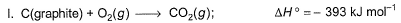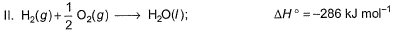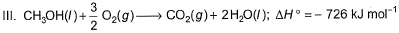Q. Thus, heat of formation of CH3OH(/)is

Solution:

Let's number our eqns-
CH3OH + 3/2 O2 → CO2 + 2H2O ...(1)
ΔH1 = –726 kJ/mol
C + O2 → CO2 ...(2)
ΔH2 = –393 kJ/mol
H2 + 1/2 O2 → H2O ...(3)
ΔH3 = –286 kJ/mol
Eqn(2) + 2×eqn(3) - eqn(1) :-
C + O2 + 2H2 + O2 + CO2 + 2H20→ CO2 + 2H20 + CH3OH + 3/2 O2
C + 1/2 O2 + 2H2 → CH3OH
Thus enthalpy of formation-
∆Hf(CH3OH) = ∆H2 + 2∆H3 - ∆H1
∆Hf(CH3OH) = -393 + 2(-286) + 726
∆Hf(CH3OH) = -239 kJ/mol

QUESTION: 5

Hot carbon reacts with steam to produce an equimolar mixture of CO(g)and H2(g) known as water gas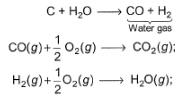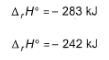Q.
Energy released , if water gas is used as fuel is

Solution: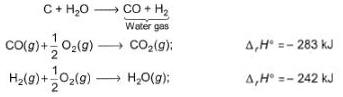For this, we will add combustion reactions of both CO and H2 as both combined will give us energy.
ΔrH = -283 kJ+ (-242 kJ) = -525 kJ

QUESTION: 6

For a gaseous phase reaction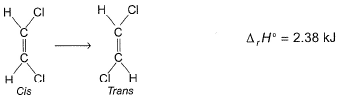When equilibrium is set up, K = 0.33
Energy involved (in kJ) is

Solution:
QUESTION: 7

One mole of a non-ideal gas undergoes a change of state (2.0 atm, 3.0 L, 95 K) → (4.0 atm, 5.0 L, 245 K) with a change in internal energy, ΔrE° = 30.0 L atm. The change in enthalpy (ΔrH°) of the process in L-atm is

[IIT JEE 2002]

Solution:

ΔH=ΔU+Δ(PV)
ΔH=30+(P2V2−P1V1)
ΔH=30+(20−6)
ΔH=30+14=44

QUESTION: 8

Which of the following reactions defines ΔfH° ?

Solution:

For ∆H formation we have to look for following:
1. formation of "1 mole" of substance.
2. from "stable" constituting elements.

In first option 1 mole of Co2 should be formed from C(graphite) for enthalpy of formation to be defined.
In third option 2 moles of NH3 is formed instead of 1 mole. So incorrect.
In fourth option Co2 is not formed from constituent elements I.e C(gr) and O2(g) so it is not enthalpy of formation.

QUESTION: 9

Ethanol can undergo decomposition to form two sets of products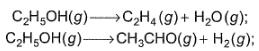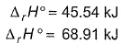Q.
if the molar ratio of C2H4 to CH3CHO is 8 : 1 in a set of product gases, then, energy involved in the decomposition process is

Solution:

A/Q molar ratio of C2H4 and CH3CHO is 8:1
So from eqn 1
∆H = 8 45.54
= 364.32 kJ
Similarly from eqn
∆H = 68.91 1
= 68.91 kJ
Total ∆H = 364.32+68.91
Therefore, enthalpy change per mole of C2H5OH used 364.32+68.91/9
= 48.14 kJ

QUESTION: 10

Direction (Q. No. 10) This sectionis based on statement I and Statement II. Select the correct answer from the code given below.

Q.

Statement I : Based on the following thermodynamic data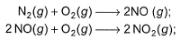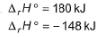NO2 is more stable than NO.

Statement II : NO (g) is an endothermic compound while, NO2(g) is an exothermic compound.

Solution:

Reaction number 1 is less stable because heat is not released thus the product side of reaction gains energy which get stored in the product and decreases its stability
While in reaction number 2, the reaction formed is more stable because energy is released and so the product is in a more stable state.
Now there is no such endothermic or exothermic compound , the reaction is exothermic or endothermic and not the product.

*Multiple options can be correct
QUESTION: 11

Direction (Q. Nos. 11-12) This section contains 2 multiple choice questions. Each question has four choices (a), (b), (c) and (d), out of which ONE or  MORE THANT ONE  is correct.

Given,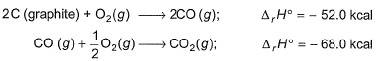Q. Thus, standard heat of formation of

Solution:
*Multiple options can be correct
QUESTION: 12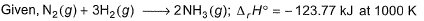Thus,

Solution:

The correct answer is option C
From, Kirchhoff's equation
△H2​ (1000 K) = △H1​(300 K) + △Cp​(1000−300)
Here, △H2​(1000 K) = −123.77 kJ mol−1
△H1​(300 K)=?
△Cp​ = 2Cp​(NH3​) − [Cp​(N2​) + 3Cp​(H2​)]
=−6R
=−6 × 8.314 × 10−3kJ
∴−123.77 = △H1​(300 K) + 6 × 8.314 × 10−3× 700
or △H1​(300 K) = −88.85 kJ
For two moles of NH3​
∴△Hf​ (NH3​) = △H1​(300K)/2​
=−88.85/​2
=−44.42 kJ mol−1

QUESTION: 13

Direction (Q. Nos. 13-15) This section contains a paragraph, wach describing  theory, experiments, data etc. three Questions related to paragraph have been  given.Each question have only one correct answer among the four given  ptions  (a),(b),(c),(d).

Based on the following thermodynamic data,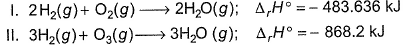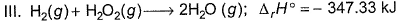Q. Which oxidising agent will generate the greatest amount of energy per mole of H2(g)?

Solution:
QUESTION: 14

Based on the following thermodynamic data,Q. Which oxidising agent will generate the greatest amount of energy per gram of oxidising agent?

Solution: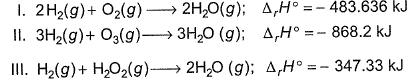Mass of O2=32 gm ∆H/gm = -483.636/32 = -15.11
Mass of O3=48 gm ∆H/gm = -868.2/48 = -18.08
Mass of H2O2=34 gm, ∆H/gm = -347.33/34 = -10.21
We can say that II has highest amount of energy per gram of oxidising agent.

QUESTION: 15

Based on the following thermodynamic data,Q. On the total mass basis of reactants, which reaction will generate the greatest amount of heat?

Solution: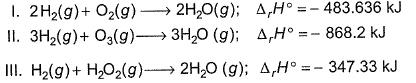From I, total mass of reactant, 36 gm
So, ∆H/mass = -483.636/36 = -13.43 kJ gm-1
For II, total mass of reactant, 54 gm
So, ∆H/mass = -868.2/54 = -16.07 kJ gm-1
FOr III, total mass = 36 gm,
So, ∆H/mass = -347.33/36 = -9.64 kJ gm-1
Therefore, heat released per unit mass is maximum for II.

*Answer can only contain numeric values
QUESTION: 16

Direction (Q. Nos. 16 - 18) This section contains 3 questions. when worked out will result in an integer from 0 to 9 (both inclusive).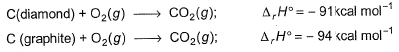Q. How much heat (in kcal) is required to convert 36 g of diamond into graphite?

Solution:

C(diamond) + O2(g) → CO2(g) -----(i)     ∆rH°  = -91 kcal mol-1
C(graphite) + O2(g) → CO2(g) -----(ii)     ∆rH°  = -94 kcal mol-1
For 36 gm of C(diamond), we have 3 moles of diamond,
On (i)-(ii), we get → C(graphite)  ∆rH°  =-91-( -94 ) kcal mol-1 = 3 kcal mol-1
So for 3 moles, we have 3×3 = 9 kcal
C(diamond)

*Answer can only contain numeric values
QUESTION: 17

Based on the following reactions,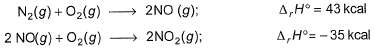Q. Heat of formation of NO2 (in kcal) is ........

Solution:

N2(g) + O2(g) → 2NO ----- (i) ∆rH° = 43 kcal
2NO(g) + O2(g) → 2NO2(g) -----(ii)     ∆rH° = -35 kcal
Enthalpy of formation of NO2 means we need to have 1 mole of NO2 from its constituting elements.
We will have (i)/2 + (ii)/2
½ N2(g) + O2(g) → NO2(g) ∆fH° = 4 kcal

*Answer can only contain numeric values
QUESTION: 18

How many of the following has zero standard molar enthalpy of the formation at 298 K?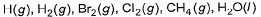[IIT JEE 2010]

Solution:

The standard molar enthalpy of formation of elements in its standard state is taken as zero. Since the standard state of H2 and Cl2 are gas, their ΔfHo = 0.